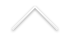O evento ocorrerá na UFF, na auditório do Instituto de Matemática e Estatística (IME), no dia 25 de novembro, a partir das 14h. A programação incluirá três palestras:

14h - 14h50: Hitoshi Ishii (Tsuda University)
Título: Two asymptotic problems for Hamilton-Jacobi equations and weak KAM theory
Resumo: In the talk, I discuss two asymptotic problems concerning Hamilton-Jacobi equations. One concerns the long-time behavior of solutions of time evolutionary Hamilton-Jacobi equations and the other is the so-called vanishing discount problem for stationary Hamilton-Jacobi equations. The last two decades have seen a fundamental importance of weak KAM theory in the asymptotic analysis of Hamilton-Jacobi equations. I explain briefly the Aubry sets and Mather measures from weak KAM theory and their use in the analysis of the two asymptotic problems above.

14h50 - 15h40: Roberto Guglielmi (EMAP-FGV)
Título: Indirect stabilization of hyperbolic systems
Resumo: We investigate stability properties of systems of hyperbolic equations, with coupling and damping terms acting either on the boundary of the domain or distributed in it. We study systems where only one component is damped, while the other equation is indirectly stabilized through the coupling with the first component. We first show that uniform exponential stability cannot hold for the whole system, thus weaker decay rates should be sought for. Therefore, by means of energy-type methods, we prove polynomial decay of the energy of solutions, linking the decay rate to the regularity of the initial conditions.

16h00 - 16h50: Juliana Fernandes (UFRJ)
Título: Semilinear parabolic equations with asymptotically linear growth
Resumo: We present some recent work on the existence and behaviour of solutions for a class of semilinear parabolic equations, defined on a bounded smooth n-dimensional domain, and we assume that the nonlinearity is asymptotically linear at infinity. We analyze the behavior of the solutions when the initial data varies in the phase space. We obtain global solutions which may be bounded or blowup in infinite time (grow-up). Our main tools are the comparison principle and variational methods. Particular attention is paid to initial data at high energy level. We use the Nehari manifold to separate the phase space into regions of initial data where uniform boundedness or grow-up behavior of the semiflow may occur. This is based on a joint work with L. Maia.